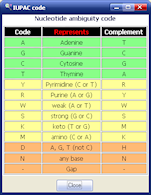Nucleotide ambiguity code

(IUPAC)

Nucleotide ambiguity code

(as defined in DNA Sequence Assembler)

 Code Represents Complement A Adenine T G Guanine C C Cytosine G T Thymine A Y Pyrimidine (C or T) R R Purine (A or G) Y W weak (A or T) W S strong (G or C) S K keto (T or G) M M amino (C or A) K D A, G, T (not C) H V A, C, G (not T) B H A, C, T (not G) D B C, G, T (not A) V X/N any base X/N - Gap -

#Code example:

Restriction enzyme: AarI

Recognition site: CACCTGCNNNN'NNNN_

Cleavage of DNA (/):

5'- C A C C T  G C N N N N/N N N N -3'
3'- G T G G A C G N N N N N N N N/-5'

The letter codes and compliment translations are those proposed by Nomenclature Committee of the International Union of Biochemistry and Molecular Biology (NC-UIBMB)

Note:

DNA Sequence Assembler can automatically detect SNP and convert them to IUPAC codes. Its Reverse Complement tool can also automatically reverse complement IUPAC bases.Standard Ambiguity Codes

The standard ambiguity codes for nucleotides and for the one-letter and three-letter designations of amino acids are given. The synonymous codons for the amino acids, and their depiction in IUB codes (Nomenclature Committee, 1985, Eur. J. Biochem. 150:1-5) are also shown.

 Nucleotide Symbol 3-Let Amino Acid IUB (Adenosine) A A Ala Alanine GCX C or G or T/U B Asx Aspartate or Asparagine RAY (Cytidine) C C Cys Cysteine UGY A or G or T/U D Asp Aspartate GAY - E Glu Glutamate GAR - F Phe Phenylalanine UUY (Guanosine) G G Gly Glycine GGX A or C or T/U H His Histidine CAY (Inosine) I I Ile Isoleucine AUH - J - - - G or T/U K Lys Lysine AAR - L Leu Leucine UUR,CUX,YUR A or C M Met Methionine AUG unknown base N Asn Asparagine AAY - O - - - - P Pro Proline CCX - Q Gln Glutamine CAR (Purine) A or G R Arg Arginine CGX,AGR,MGR C or G S Ser Serine UCX,AGY (Thymidine) T T Thr Threonine ACX (Uridine) U U - - - A or C or G V Val Valine GUX A or T/U W Trp Tryptophan UGG unknown base X unknown amino acid XXX (Pyrimidine) C or T/U Y Tyr Tyrosine UAY - Z Glx Glutamate or Glutamine SAR no base (deletion/gap) . no amino acid (deletion/gap) - - - * End terminator UAR,URA

 How the standard ambiguity codes were assigned Standard Amino Acid Codes A = Ala = Alanine C = Cys =Cysteine (not Cystine!) G = Gly = Glycine I = Ile =Isoleucine L = Leu = Leucine M = Met = Methionine P = Pro = Proline S = Ser = Serine T = Thr = Threonine V = Val = Valine should be obvious codes Standard Nucleotide Codes A = Adenylic acid C = Cytidylic acid G = Guanylic acid T = Thymidylic acid U = Uridylic acid I = Inosylic acid       should be obvious codes F = Phe = Phenylanine N = Asn = Asparagine R = Arg = Arginine Y = Tyr = Tyrosine   are phonetic codes R = A or G = puRine Y = C or T = pYrimidine K = G or T = Keto M = A or C = aMino S = G or C = Strong base pair W = A or T = Weak base pair double base codes D = Asp = Aspartic acid E = Glu = Glutamic acid K = Lys = Lysine Q = Gln = Glutamine W = Trp = Tryptophan (big letter big residue) non-obvious codes (you just have to learn them!) B = not A (G or C or T) D = not C (A or G or T) H = not G (A or C or T) V = not T/U (A or C or G)   triple base codes B = Asx = Aspartic acid or Asparagine Z = Glx = Glutamic acid or Glutamine   these are ambiguity codes N = aNy base  (by convention, X is used for unknown amino acids, N for unknown nucleotides) X = any amino acid J, O, U = no amino acid codes .(dot) = deletion or gap *(star) = End or terminator E, F, J, L, O, P, Q, Z have no base codes .(dot) = deletion or gap